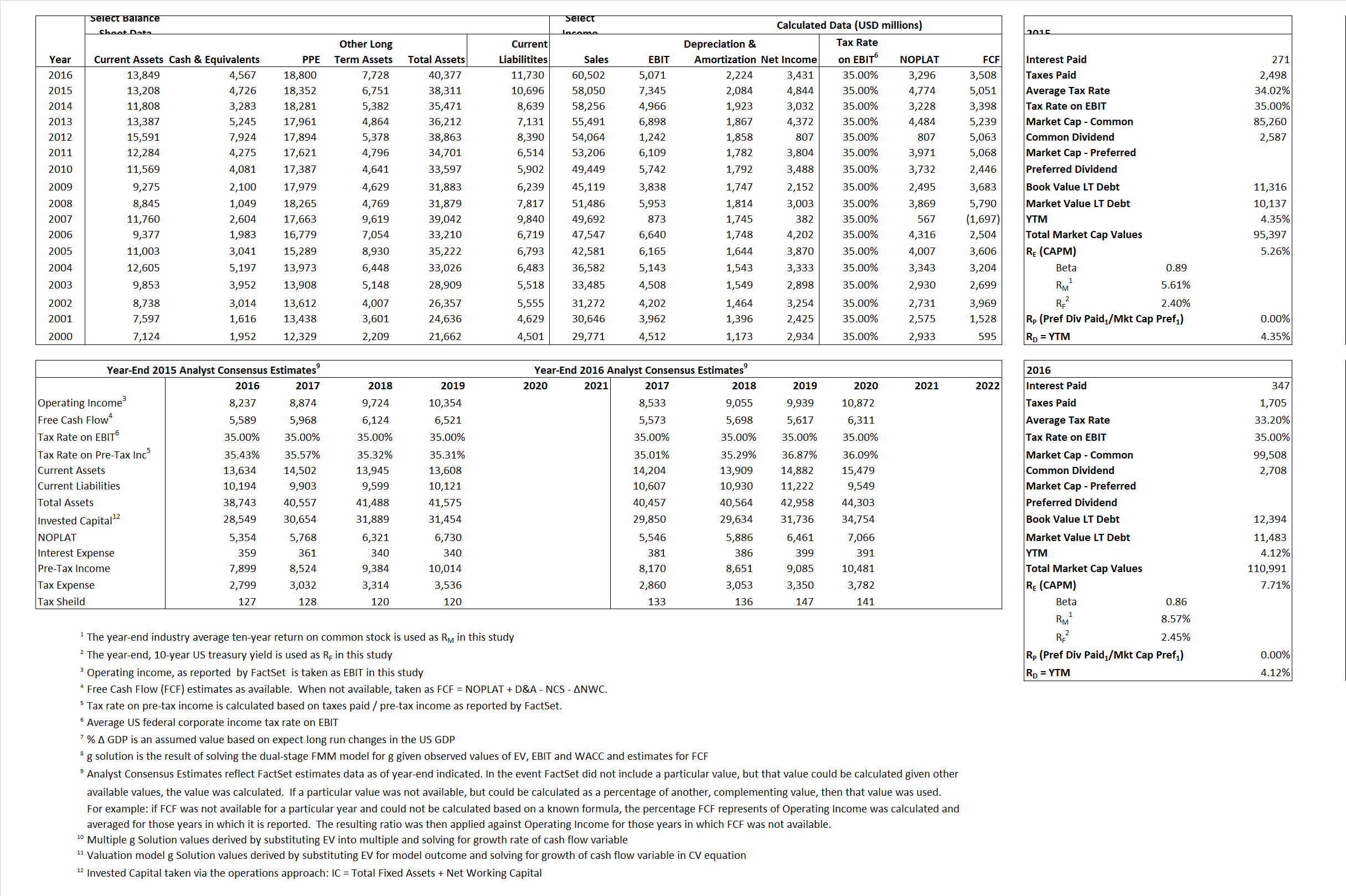# United Parcel Service Inc

## Analyst Listing

The following analysts provide coverage for the subject firm as of May 2016:

 Broker Analyst Analyst Email Credit Suisse Allison M. Landry allison.landry@credit-suisse.com Raymond James Arthur W. Hatfield art.hatfield@raymondjames.com Susquehanna Financial Group Bascome Majors bascome.majors@sig.com Stifel Nicolaus David G. Ross dross@stifel.com Bernstein Research David Vernon david.vernon@bernstein.com Avondale Partners Donald Broughton dbroughton@avondalepartnersllc.com Cowen & Company Helane Becker helane.becker@cowen.com Stephens Inc Jack Atkins jack.atkins@stephens.com Buckingham Research Jeffrey Kauffman jkauffman@buckresearch.com RBC Capital Markets John Barnes john.barnes@rbccm.com BB&T Capital Markets Kevin W. Sterling ksterling@bbandtcm.com Nomura Research Matt Troy matt.troy@nomura.com William Blair Nathan Brochmann nbrochmann@williamblair.com Deutsche Bank Research Robert Salmon robert.salmon@db.com Wolfe Research Scott H. Group sgroup@wolferesearch.com Oppenheimer Scott Schneeberger scott.schneeberger@opco.com

## Primary Input Data## Derived Input Data

### Equational Form

Net Operating Profit Less Adjusted Taxes NOPLAT  4,774 3,296$NOPLAT\, =\, EBIT\, x\, (1 \,-\, Avg \,\,Tax\,\, Rate\,\, on\,\, EBIT)$
Free Cash Flow FCF 5,051 4,774$FCF\,=NOPLAT\,+\,Non-Cash\,Expenses-\Delta NWC\,-\,NCS$
Tax Shield TS 92 115$TS\,=\,Interest\,\,Paid\,\,x\,\, Avg \,\,Tax\,\,Rate\,\, on\,\, Pre-Tax\,\, Income$
Invested Capital IC 27,615 28,647$IC\,=\,Fixed\,\,Operating\,\,Assets\,\,+\,\,Net\,\, Working\,\, Capital$
Return on Invested Capital ROIC 17.29% 11.51%$ROIC\,=\,\frac { NOPLAT }{ IC }$
Net Investment NetInv 2,867 3,256$NetInv\,=\,{ {IC}_{1}}-{{IC}_{0}}+Depreciation$
Investment Rate IR 60.05% 98.78%$IR\,=\,\frac {NetInv}{NOPLAT}$
Weighted Average Cost of Capital
WACCMarket 5.00% 7.20%$WACC\,=\,\frac { E }{ V } { R }_{ E }\,+\,\frac { P }{ V } { R }_{ P }\,+\,\frac { D }{ V } { R }_{ D }\left( 1- Avg\,\, Tax\,\,Rate\,\,on\,\,Pre-Tax\,\,Income \right)$
WACCBook  7.62%  7.17%
Enterprise value
EVMarket 90,671 106,424$EV\,=\,Market\,\,Cap\,\,Equity\,+\,\,Long\,\,Term\,\,Debt\,-\,Cash$
EVBook  92,009  107,335
Long-Run Growth
g = IR x ROIC
10.38% 11.37% Long-run growth rates of the income variable are used in the Continuing Value portion of the valuation models.
g = %$\Delta$ GDP  2.50%  2.50%
Margin from Operations M 12.65% 8.38%$M\,\,=\,\,\frac{EBIT}{SALES}$
Depreciation/Amortization Rate D 22.10% 30.49%$D\,\,=\,\,\frac{D+A}{EBITDA}$

## Valuation Multiple Outcomes

The outcomes presented in this study are the result of original input data, derived data, and synthesized inputs.

### model g solution

12/31/2015 12/31/2016 12/31/2015 12/31/2016 12/31/2015 12/31/2016

EV/SALES$\frac {EV}{Sales} \,= \,\frac{ROIC\, -\, g}{ROIC\,(WACC\,-\,g)}\,(1\,-\,T)\,(M)$

1.56  1.76  -0.38%  5.61%  -0.99%  6.10%

EV/EBITDA$\frac {EV}{EBITDA} \,= \,\frac{ROIC\, -\, g}{ROIC\,(WACC\,-\,g)}\,(1\,-\,T)\,(1\,-\,D)$

9.62 14.59 -0.38% 5.61% -0.99%  6.10%

EV/NOPLAT$\frac {EV}{NOPLAT} \,= \,\frac{ROIC\, -\, g}{ROIC\,(WACC\,-\,g)}$

18.99 32.29 -0.38% 5.61% -0.99%  6.10%

EV/FCFOPS$\frac {EV}{FCF_{OPS}} \,= \,\frac{ROIC\, -\, g}{ROIC\,(WACC\,-\,g)}\,(1\,-\,T)$

17.95 30.34 -0.38% 5.61% -0.99%  6.10%

EV/EBIT$\frac {EV}{EBIT} \,= \,\frac{ROIC\, -\, g}{ROIC\,(WACC\,-\,g)}\,(1\,-\,T)$

12.34 20.99 -0.38% 5.61% -0.99%  6.10%

EV/IC$\frac {EV}{IC} \,= \,\frac{ROIC\, -\, g}{WACC\,-\,g}$

3.28 3.72 -0.38% 5.61% -0.99%  6.10%